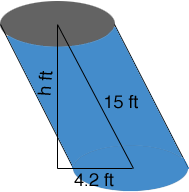SEARCH HOMEMath Central Quandaries & QueriesQuestion from tom, a student: The outer protective smokestack of a steamship is streamlined so that it has uniform oval section parallel to the deck. The area of this oval section is 48 sq. ft . If the length of the stack is 15 ft. and the stack is raked aft so that its axis at its upper end is horizontally 4.2 ft from the lower end, find the volume enclosed by the stack. Provided that the area of the section is given and the length(element) as well, then I can calculate the volume...but why it doesn't match the the answer ? I really need to see what it looks like...will you? pls?Hi Tom,

Here is my diagram of the smokestack.The volume is the area of the base (section) times the height h. Does this help?

PennyMath Central is supported by the University of Regina and The Pacific Institute for the Mathematical Sciences.